## The SpaceR2

Algebraically, the familiar x−y plane is simply the collection of all pairs ( x, y) of real numbers. Each such pair specifies a point in the plane as follows. First, construct two copies of the real line—one horizontal and one vertical—which intersect perpendicularly at their origins; these are called the axes. Then, given a pair (x 1, x 2), the first coordinate, x 1, specifies the point's horizontal displacement from the vertical axis, while the second coordinate, x 2, gives the vertical displacement from the horizontal axis. See Figure 1. Clearly, then, the order in which the coordinates are written is important since the point (x 1, x 2) will not coincide—generally—with the point (x 2, x1). To emphasize this fact, the plane is said to be the collection of ordered pairs of real numbers. Since it takes two real numbers to specify a point in the plane, the collection of ordered pairs (or the plane) is called 2‐space, denoted R 2 (“R two”).Figure 1

R 2 is given an algebraic structure by defining two operations on its points. These operations are addition and scalar multiplication. The sum of two points x=(x 2, x 1) and x′=(x′ 1, x′ 2) is defined (quite naturally) by the equation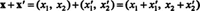and a point x=(x 1, x 2) is multiplied by a scalar c (that is, by a real number) by the rule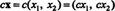Example 1: Let x = (1, 3) and y = (−2, 5). Determined the points x + y, 3x, and 2x − y.

The point x + y is (1, 3) + (−2, 5) = (−1, 8), and the point 3x equals 3(1, 3) = (3, 9). Since −y = (−1)y = (2, −5),By defining x − x′ to be x + (−x′), the difference of two points can be given directly by the equationThus, the point 2x − y could also have been calculated as follows:Vectors in R 2 . A geometric vector is a directed line segment from an initial point (the tail) to a terminal or endpoint (the tip). It is pictured as an arrow as in Figure 2.Figure 2

The vector from point a to point b is denoted ab. If a=(a 1, a 2) is the initial point and b = (b 1, b2) is the terminal point, then the signed numbers b 1,− a 1 and b 2,− a 2 are called the components of the vector ab. The first component, b 1,− a 1, indicates the horizontal displacement from a to b, and the second component, b 2,− a 2 indicates the vertical displacement. See Figure 3 . The components are enclosed in parentheses to specify the vector, thus, ab = (b 1 − a 1, b 2 − a 2).Figure 3

Example 2: If a = (4, 2) and b = (−5, 6), then the vector from a to b has a horizontal component of −5 − 4 = −9 and a vertical component of 6 − 2 = 4. Therefore, ab = (−9, 4), which is sketched in Figure 4.Figure 4

Example 3: Find the terminal point of the vector xy = (8, −7) if its initial point is x = (−3, 5).

Since the first component of the vector is 8, adding 8 to the first coordinate of its initial point will give the first coordinate of its terminal point. Thus, y 1 = x + 8 = −3 + 8 = 5.Similarly, since the second component of its initial point will give the second coordinate of its endpoint. This gives y 2 = x 2 + (−7)= 5 + (−7)= −2. The terminal point of the vector xy is therefore y = (y 1, y2) = (5, −2); see Figure 5.Figure 5

Two vectors in R 2 are said to be equivalent (or equal) if they have the same first component and the same second component. For instance, consider the points a = (−1, 1), b = (1, 4), c = (1, −2), and d (3, 1). The horizontal component of the vector ab is 1 − (−1) = 2, and the vertical component of ab is 4 − 1 = 3; thus, ab = (2, 3). Since the vector cd has a horizontal component of 3 − 1 = 2, and a vertical component of 1 − (−2) = 3, cd = (2, 3) also. Therefore, ab = cd; see Figure 6.Figure 7

To translate a vector means to slide it so as to change its initial and terminal points but not its components. If the vector ab in Figure were translated to begin at the point c = (1, −2), it would coincide with the vector cd. This is another way to say that ab = cd.

Example 4: Is the vector from a = (0, 2) to b = (3, 5) equivalent to the vector from x = (2, −4) to y = (5, 1)?

Since the vector ab equals (3, 3), but the vector xy equals (3, 5), these vectors are not equivalent. Alternatively, if the vector ab were translated to begin at the point x, its terminal point would then be ( x + 3, x + 3) = (5, −1). This is not the point y; thus, ab ≠ xy.

Position vectors. If a vector has its initial point at the origin, the point 0 = (0, 0), it is called a position vector. If a position vector has x = (x 1, x2), as its endpoint, then the components of the vector 0x are x 1 − 0 = x 1 and x 2 − 0 = x 2; so 0x = (x 1, x 2). If the origin is not explicitly written, then a position vector can be named by simply specifying its endpoint; thus, x = (x 1, x2). Note that the position vector x with components x 1 and x 2 is denoted (x 1, x2), justlike the point x with coordinates x 1 and x 2. The context will make it clear which meaning is intended, but often the difference is irrelevant. Furthermore, since a position vector can be translated to begin at any other point in the plane without altering the vector (since translation leaves the components unchanged), even vectors that do not begin at the origin are named by a single letter.

Example 5: If the position vector x = (−4, 2) is translated so that its new initial point is a = (3, 1), find its new terminal point, b.

If b = (b 1, b2), then the components of the vector ab are b 1 − 3 and b 2 − 1. Since ab = x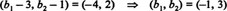See Figure 7.Figure 7

Vector addition. The operations defined earlier on points (x 1, x2) in R 2 can be recast as operations on vectors in R 2 (called 2‐vectors, because there are 2 components). These operations are called vector addition and scalar multiplication. The sum of two vectors x and x′ is defined by the same rule that gave the sum of two points:Figure depicts the sum of two vectors. Geometrically, one of the vectors (x′, say) is translated so that its tail coincides with the tip of x. The vector from the tail of x to the tip of the translated x′ is the vector sum x + x′. This process is often referred to as adding vectors tip‐to‐tail.Figure 8

Because the addition of real number is commutative, that is, because the order in which numbers are added is irrelevant, it follows that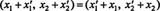This implies the addition of vectcrs is commutative also: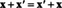Thus, when adding x and x′ geometrically, it doesn't matter whether x′ is first translated to begin at the tip of x or x is translated to begin at the tip of x′; the sum will be the same in either case.

Example 6: The sum of the vectors x = (1, 3) and y = (−2, 5) is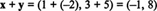See Figure 9.Figure 9

Example 7: Consider the position vector a = (1, 3). If b is the point (5, 4), find the vector ab and the vector sum a + ab. Provide a sketch.

Since ab has horizontal component 5 − 1 = 4 and vertical component 4 − 3 = 1, the vector ab equals (4, 1). So a + ab = (1, 3) + (4, 1) = (5, 4), which is the position vector b. Figure 10 clearly shows that a + ab = b.Figure 10

Vector subtraction. The difference of two vectors is defined in precisely the same way as the difference of two points. For any two vectors x and x′ in R 2,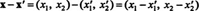With x and x′ starting from the same point, x − x′ is the vector that begins at the tip of x′ and ends at the tip of x. This observation follows from the identity x′ + (x − x′) = x and the method of adding vectors geometrically. See Figure 11 .Figure 11

In general, it is easy to see that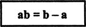whether the letters on the right‐hand side are interpreted as position vectors or as points. Figure showed that a + ab = b, which is equivalent to the statement ab = b − a, where a and b are position vectors. Although this example dealt with a particular case, the identity ab = b − a holds in general.

Example 8: The vector ab from a = (4,−1) to b = (−2, 1) in Figure 12 isFigure 12

Scalar multiplication. A vector x is multiplied by a scalar c by the ruleIf the scalar c is 0, then for any x, c x equals (0, 0)—the zerovector, denoted 0. If c is positive, the vector c x points in the same direction as x, and it can be shown that its length is c times the length of x. However, if the scalar c is negative, then c x points in the direction exactly opposite to that of the original x, and the length of c x is |c| times the length of x. Some examples are shown in Figure 13:Figure 13

Two vectors are said to be parallel if one is a positive scalar multiple of the other and antiparallel if one is a negative scalar multiple of the other. (Note: Some authors declare two vectors parallel if one is a scalar multiple—positive or negative—of the other.)

Standard basis vectors in R 2 . By invoking the defintions of vector addition and scalar multiplication, any vector x = (x 1, x 2) in R 2 can be written in terms of the standard basis vectors (1, 0) and (0, 1):The vector (1, 0) is denoted by i (or e 1), and the vector (0, 1) is denoted by j (or e 2). Using this notation, any vector x in R 2 can be written in either of the two forms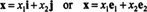See Figure 14.Figure 14

Example 9: If x = 2 i + 4 j and y = i − 3 j, determine (and provide a sketch of) the vectors 1/2 x and x + y.

Multiplying the vector x by the scalar 1/2 yieldsThe sum of the vectors x and y is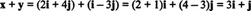These vectors are shown (together with x and y) in Figure 15.Figure 15

Example 10: Find the scalar coefficients k 1 and k 2 such that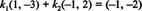The given equation can be rewritten as follows:This implies that both of the following equations must be satisfied: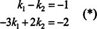Multiplying the first equation by 3 then adding the result to the second equation yields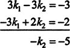Thus, k 2 = 5. Substituting this result back into either of the equations in (*) gives k 1 = 4.